# High School Math : How to find the area of an equilateral triangle

## Example Questions

2 Next →

### Example Question #11 : How To Find The Area Of An Equilateral Triangle

Find the area of an equilateral triangle whose perimeter is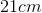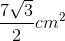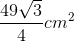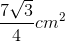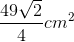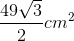Explanation:

The formula for the perimeter of an equilateral triangle is: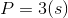Plugging in our values, we get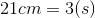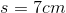The formula for the area of an equilateral triangle is: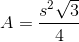Plugging in our values, we get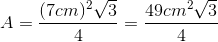### Example Question #154 : Plane Geometry

What is the area of an equilateral triangle with a side length of 5?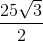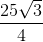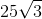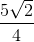Explanation:

Note that an equilateral triangle has equal sides and equal angles. The question gives us the length of the base, 5, but doesn't tell us the height.

If we split the triangle into two equal triangles, each has a base of 5/2 and a hypotenuse of 5.

Therefore we can use the Pythagorean Theorem to solve for the height: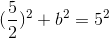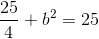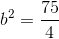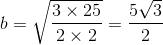Now we can find the area of the triangle: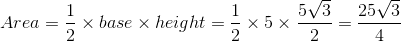### Example Question #1 : Equilateral Triangles

An equilateral triangle has a perimeter of 18. What is its area?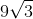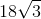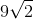Explanation:

Recall that an equilateral triangle also obeys the rules of isosceles triangles.   That means that our triangle can be represented as having a height that bisects both the opposite side and the angle from which the height is "dropped."  For our triangle, this can be represented as: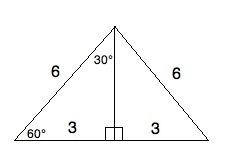Now, although we do not yet know the height, we do know from our 30-60-90 regular triangle that the side opposite the 60° angle is √3 times the length of the side across from the 30° angle. Therefore, we know that the height is 3√3.

Now, the area of a triangle is (1/2)bh. If the height is 3√3 and the base is 6, then the area is (1/2) * 6 * 3√3 = 3 * 3√3 = 9√(3).

### Example Question #163 : Plane Geometry

A circle contains 6 copies of a triangle; each joined to the others at the center of the circle, as well as joined to another triangle on the circle’s circumference.

The circumference of the circle is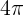What is the area of one of the triangles?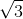Explanation:

The radius of the circle is 2, from the equation circumference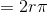. Each triangle is the same, and is equilateral, with side length of 2. The area of a triangle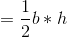To find the height of this triangle, we must divide it down the centerline, which will make two identical 30-60-90 triangles, each with a base of 1 and a hypotenuse of 2. Since these triangles are both right traingles (they have a 90 degree angle in them), we can use the Pythagorean Theorem to solve their height, which will be identical to the height of the equilateral triangle.We know that the hypotenuse is 2 so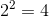. That's our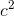solution. We know that the base is 1, and if you square 1, you get 1.

Now our formula looks like this: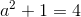, so we're getting close to finding.

Let's subtract 1 from each side of that equation, in order to make things a bit simpler: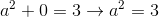Now let's apply the square root to each side of the equation, in order to change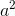into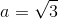Therefore, the height of our equilateral triangle isTo find the area of our equilateral triangle, we simply have to multiply half the base by the height: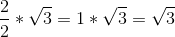The area of our triangle is2 Next →